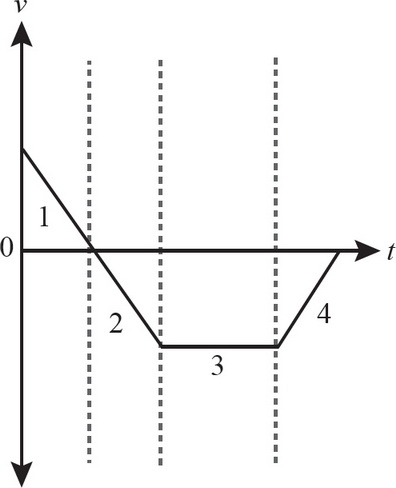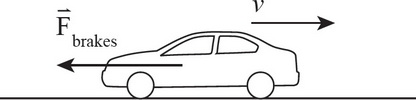# AP Physics 1 Multiple-Choice Practice Test 28

### Test Information10 questions18 minutes

1. A section of a river flows with a velocity of 1 m/s due S. A kayaker who is able to propel her kayak at 1.5 m/s wishes to paddle directly east one bank to the other. In what direction should she direct her kayak?

2. An object's initial velocity is in the second quadrant, with components v1x = -1, v1y = 4. Its final velocity is in the fourth quadrant, with components v2x = 4, v2y = -2. What is the direction of its average acceleration relative to the +x-axis?

3. A student lives 1.2 km from school. On a particular day it takes her 10 min to get from home to school. Which of the following must be true about this trip to school?

I. She travelled a distance of 1.2 km.

II. Her average speed was 2 m/s.

III. The magnitude of her average velocity was 2 m/s.

4. In which of the following position-versus-time graphs does the object in motion have a constant negative acceleration?

5. In which section of the following velocity-versus-time graph is the object speeding up and moving in the negative direction?6. An airtanker is being used to fight a forest fire. It is flying with a ground speed of 85 m/s and maintaining an altitude of 300 m. If it is flying directly towards the fire, at what approximate horizontal distance from the fire should its tanks be open in order for the water dropped to land on the fire? (Assume that the fire is relatively localized.)

7. Two cannons are fired from a cliff at a height of 50 m from the ground. Cannonball A is fired horizontally with an initial velocity of 40 m/s. Cannonball B is fired at a launch angle of 60° with an initial velocity of 80 m/s. Which cannonball will have a greater magnitude of displacement after two seconds?

8. A car initially at rest accelerates linearly at a constant rate for eight seconds. If the total displacement of the car was 600 m, what was the speed of the car after the eight seconds of acceleration?9. A car travelling at a speed of v0 applies its brakes, skidding to a stop over a distance of x m. Assuming that the deceleration due to the brakes is constant, what would be the skidding distance of the same car if the braking were twice as effective (doubling the magnitude of deceleration)?

10. A student is pushing a 3-kg book across a table with a constant force of 30.0 N directed 10° below the horizontal. The coefficient of kinetic friction between the book and the table is 0.3. What is the magnitude of the force that the table exerts on the book during this motion?